# matplotlib.artist.Artist.set#

Artist.set(*, agg_filter=<UNSET>, alpha=<UNSET>, animated=<UNSET>, clip_box=<UNSET>, clip_on=<UNSET>, clip_path=<UNSET>, gid=<UNSET>, in_layout=<UNSET>, label=<UNSET>, mouseover=<UNSET>, path_effects=<UNSET>, picker=<UNSET>, rasterized=<UNSET>, sketch_params=<UNSET>, snap=<UNSET>, transform=<UNSET>, url=<UNSET>, visible=<UNSET>, zorder=<UNSET>)[source]#

Set multiple properties at once.

Supported properties are

Property

Description

`agg_filter`

a filter function, which takes a (m, n, 3) float array and a dpi value, and returns a (m, n, 3) array and two offsets from the bottom left corner of the image

`alpha`

scalar or None

`animated`

bool

`clip_box`

`BboxBase` or None

`clip_on`

bool

`clip_path`

Patch or (Path, Transform) or None

`figure`

`Figure`

`gid`

str

`in_layout`

bool

`label`

object

`mouseover`

bool

`path_effects`

list of `AbstractPathEffect`

`picker`

None or bool or float or callable

`rasterized`

bool

`sketch_params`

(scale: float, length: float, randomness: float)

`snap`

bool or None

`transform`

`Transform`

`url`

str

`visible`

bool

`zorder`

float

## Examples using `matplotlib.artist.Artist.set`#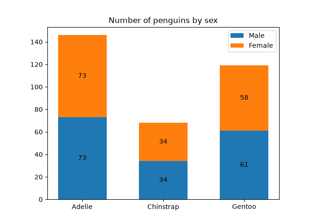Bar Label Demo

Bar Label Demo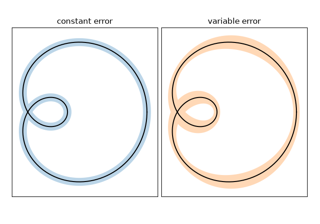Curve with error band

Curve with error band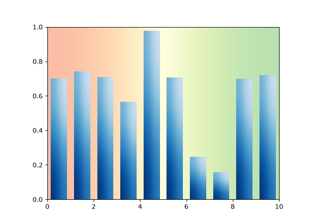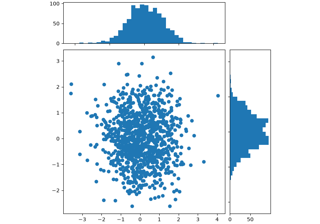Scatter plot with histograms

Scatter plot with histograms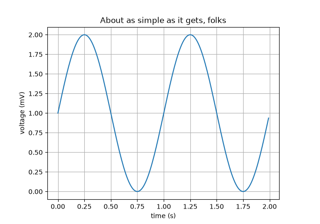Simple Plot

Simple Plot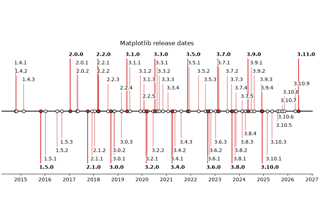Creating a timeline with lines, dates, and text

Creating a timeline with lines, dates, and text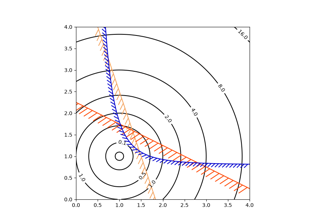Contouring the solution space of optimizations

Contouring the solution space of optimizations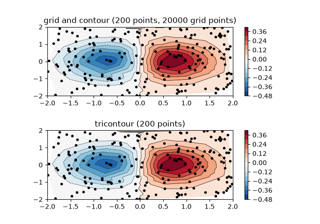Contour plot of irregularly spaced data

Contour plot of irregularly spaced data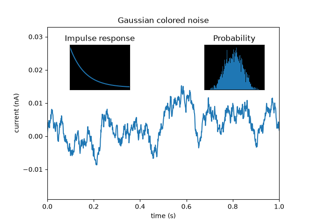Axes Demo

Axes Demo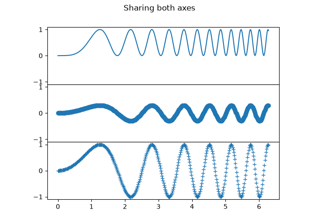Creating multiple subplots using plt.subplots

Creating multiple subplots using plt.subplots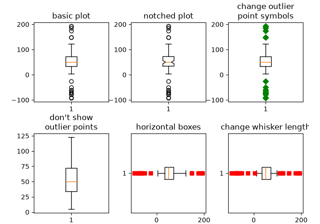Boxplots

Boxplots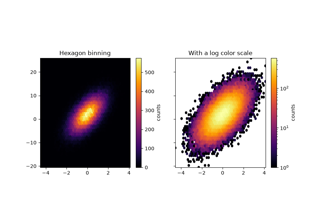Hexagonal binned plot

Hexagonal binned plot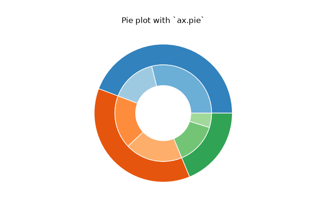Nested pie charts

Nested pie charts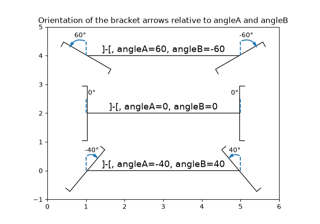Angle annotations on bracket arrows

Angle annotations on bracket arrows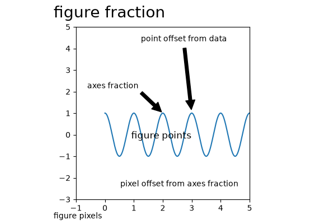Annotating Plots

Annotating Plots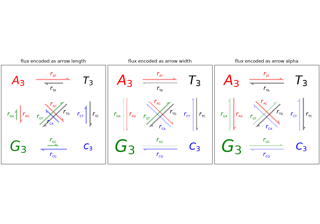Arrow Demo

Arrow Demo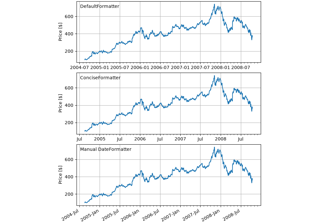Date tick labels

Date tick labels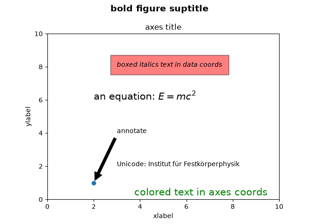Text Commands

Text Commands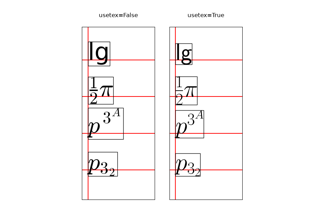Usetex Baseline Test

Usetex Baseline TestReference for Matplotlib artists

Reference for Matplotlib artists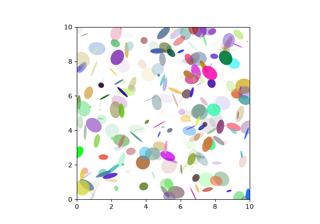Ellipse Demo

Ellipse Demo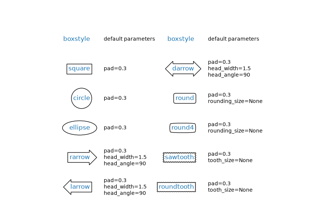Drawing fancy boxes

Drawing fancy boxes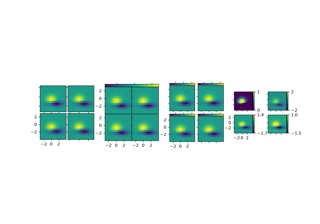Demo Axes Grid

Demo Axes GridAdding a colorbar to inset axes

Adding a colorbar to inset axes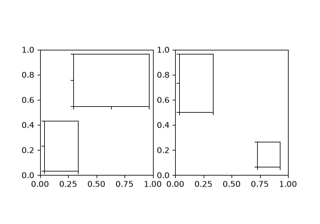Inset locator demo

Inset locator demo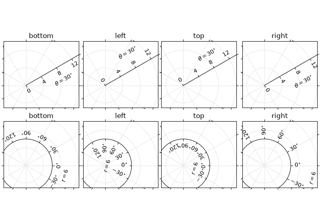axis_direction demo

axis_direction demo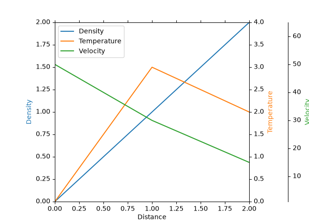Parasite Axes demo

Parasite Axes demo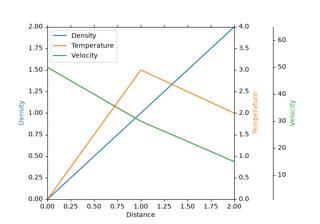Parasite axis demo

Parasite axis demo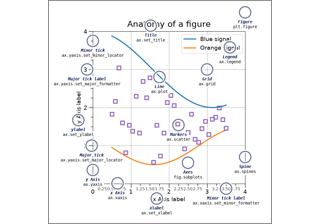Anatomy of a figure

Anatomy of a figure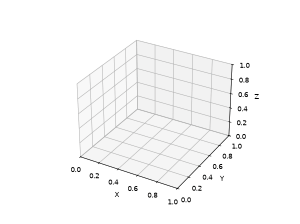Animated 3D random walk

Animated 3D random walk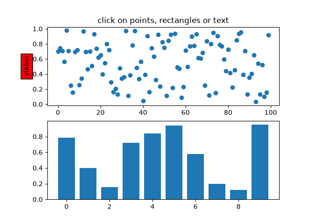Pick event demo

Pick event demo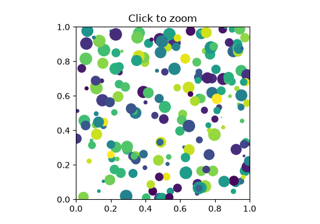Zoom Window

Zoom Window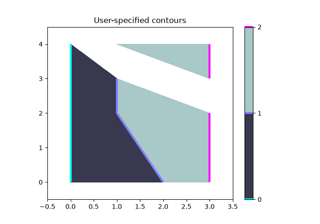Manual Contour

Manual Contour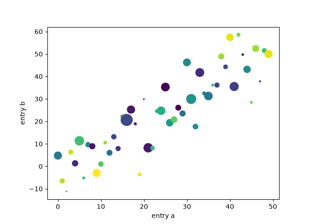Plotting with keywords

Plotting with keywords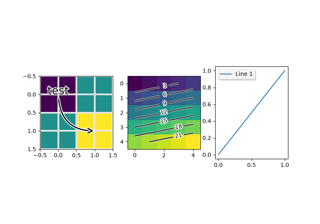Patheffect Demo

Patheffect Demo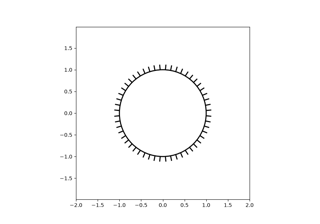TickedStroke patheffect

TickedStroke patheffect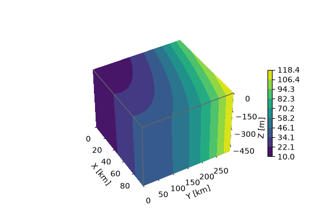3D box surface plot

3D box surface plot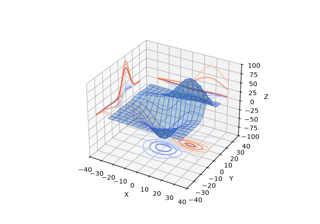Project contour profiles onto a graph

Project contour profiles onto a graph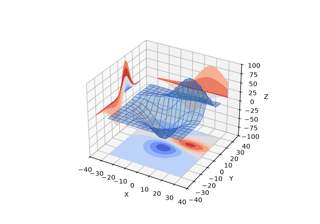Project filled contour onto a graph

Project filled contour onto a graph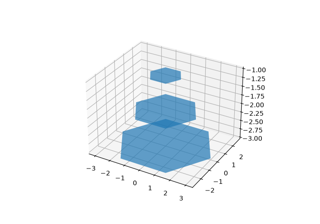Generate polygons to fill under 3D line graph

Generate polygons to fill under 3D line graph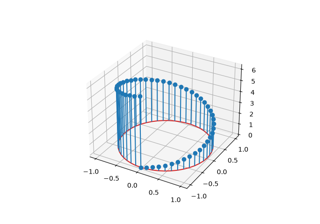3D stem

3D stem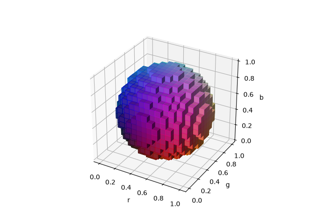3D voxel / volumetric plot with RGB colors

3D voxel / volumetric plot with RGB colors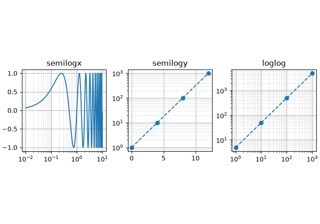Log Demo

Log Demo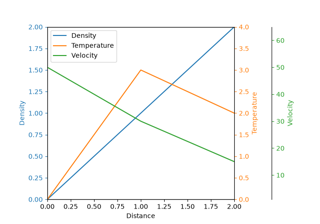Multiple y-axis with Spines

Multiple y-axis with Spines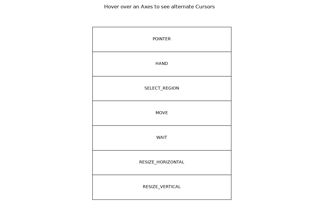Mouse Cursor

Mouse CursorAnnotate Explain

Annotate ExplainConnection styles for annotations

Connection styles for annotationsNested GridSpecs

Nested GridSpecsSimple Annotate01

Simple Annotate01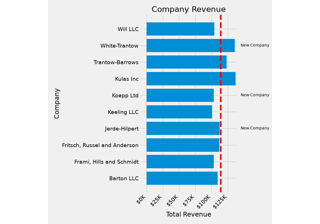The Lifecycle of a Plot

The Lifecycle of a Plot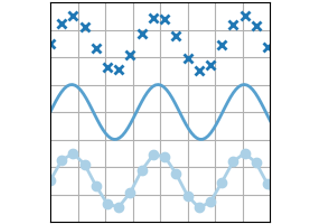plot(x, y)

plot(x, y)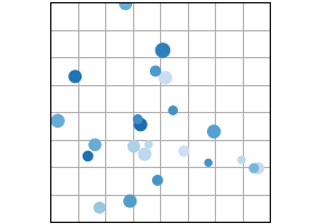scatter(x, y)

scatter(x, y)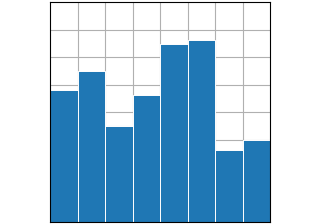bar(x, height)

bar(x, height)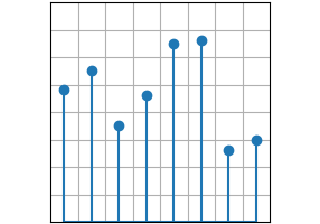stem(x, y)

stem(x, y)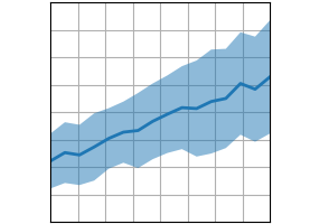fill_between(x, y1, y2)

fill_between(x, y1, y2)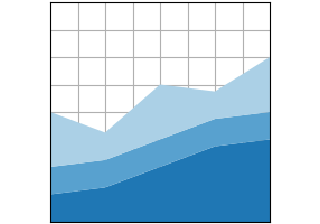stackplot(x, y)

stackplot(x, y)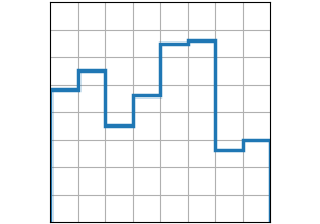stairs(values)

stairs(values)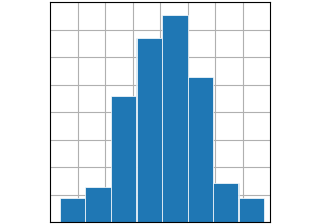hist(x)

hist(x)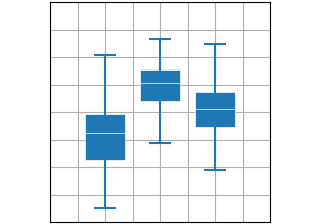boxplot(X)

boxplot(X)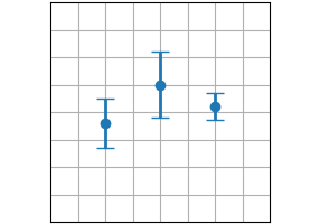errorbar(x, y, yerr, xerr)

errorbar(x, y, yerr, xerr)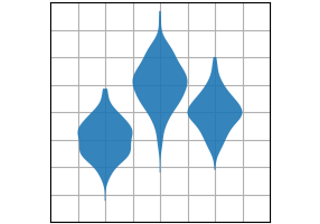violinplot(D)

violinplot(D)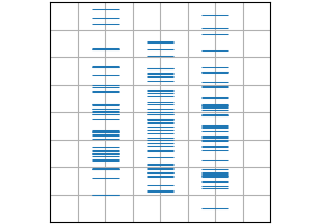eventplot(D)

eventplot(D)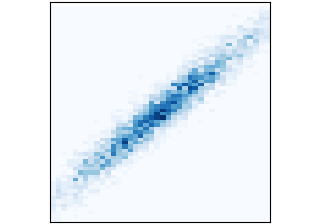hist2d(x, y)

hist2d(x, y)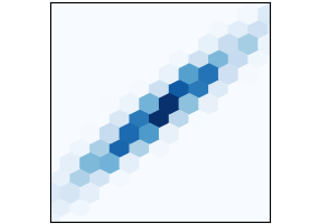hexbin(x, y, C)

hexbin(x, y, C)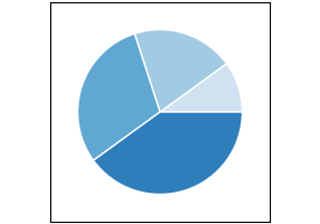pie(x)

pie(x)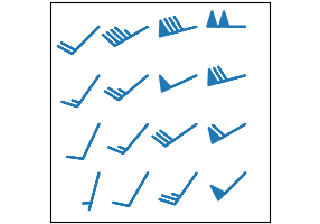barbs(X, Y, U, V)

barbs(X, Y, U, V)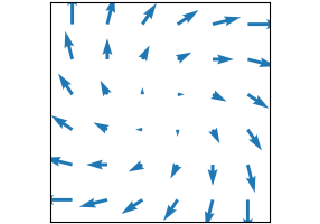quiver(X, Y, U, V)

quiver(X, Y, U, V)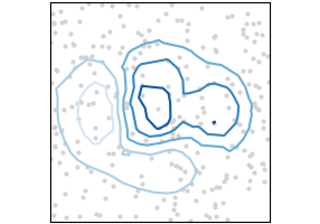tricontour(x, y, z)

tricontour(x, y, z)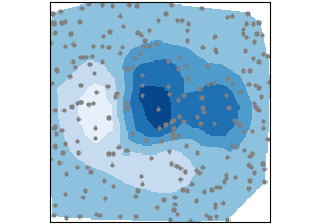tricontourf(x, y, z)

tricontourf(x, y, z)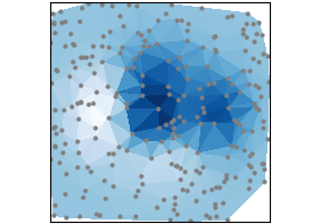tripcolor(x, y, z)

tripcolor(x, y, z)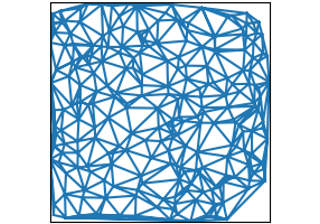triplot(x, y)

triplot(x, y)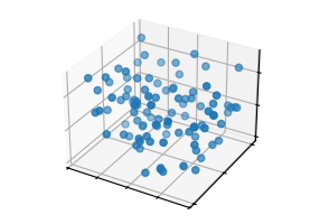scatter(xs, ys, zs)

scatter(xs, ys, zs)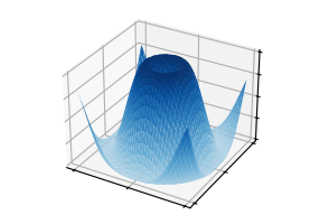plot_surface(X, Y, Z)

plot_surface(X, Y, Z)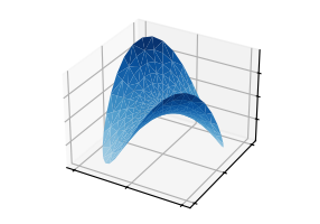plot_trisurf(x, y, z)

plot_trisurf(x, y, z)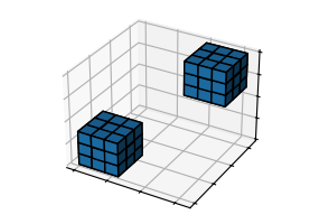voxels([x, y, z], filled)

voxels([x, y, z], filled)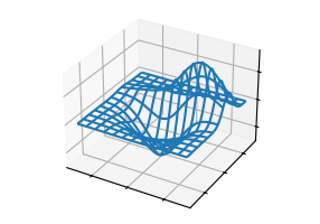plot_wireframe(X, Y, Z)

plot_wireframe(X, Y, Z)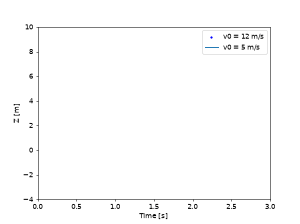Animations using Matplotlib

Animations using Matplotlib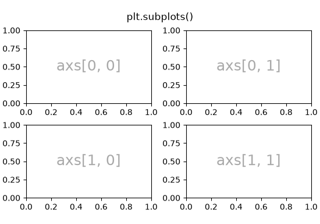Arranging multiple Axes in a Figure

Arranging multiple Axes in a Figure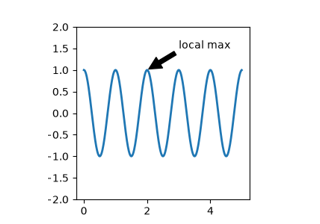Annotations

Annotations